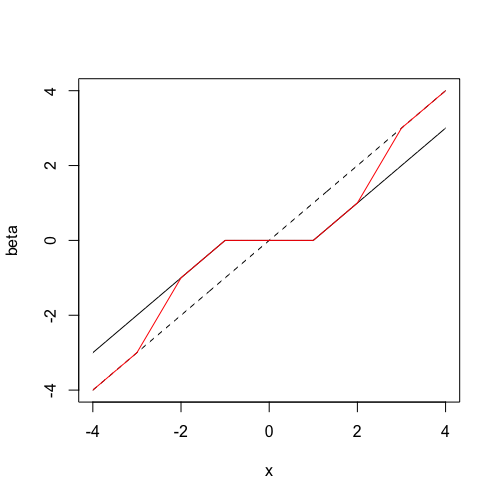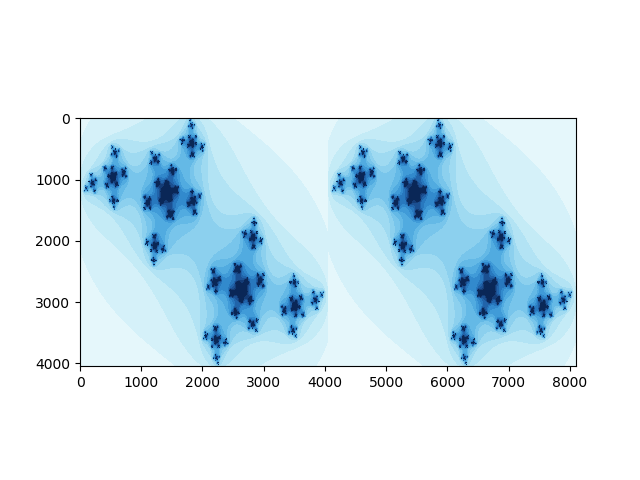新鲜事¶

pclasso¶

set.seed(1234)
n = 100; p = 10
X = matrix(rnorm(n * p), nrow = n)
y = rnorm(n)
library(pcLasso)
fit <- pcLasso(X, y, theta = 10)

predict(fit, X[1:3, ])[, 5]

groups = list(1:5, 6:10)
fit = pcLasso(X, y, theta = 10, groups = groups)

fit = cv.pcLasso(X, y, theta = 10)
predict(fit, X[1:3,], s = "lambda.min")

Emojis in scatterplot¶

References:

library(ggplot2)
library(emoGG)
data("ToothGrowth")
p1 <- geom_emoji(data = subset(ToothGrowth, supp == "OJ"),
aes(dose + runif(sum(ToothGrowth$supp == "OJ"), min = -0.2, max = 0.2), len), emoji = "1f34a") p2 <- geom_emoji(data = subset(ToothGrowth, supp == "VC"), aes(dose + runif(sum(ToothGrowth$supp == "OJ"), min = -0.2, max = 0.2),
len), emoji = "1f48a")

ggplot() +
p1 + p2 +
labs(x = "Dose (mg/day)", y = "Tooth length")

Medians in high dimensions¶

Refer to Medians in high dimensions

• marginal median
• geometric median
• medoid
• centerpoint
• Tukey median

Laplace distribution as a mixture of normal distributions¶

\int_0^\infty f_{X\mid W=w}(x)f_W(w)dw=\frac{1}{2b}\exp\Big(-\frac{\vert x\vert}{b}\Big)\,.

Gradient descent as a minimization problem¶

put gradient decent into the optimization framework, then derive

• projected gradient descent
• proximal gradient methods

Coordinate descent doesn’t always work for convex functions¶

A counterexample:

z=\max(x,y)+\vert x-y\vert

Solution to a sgn equation¶

Give a proof of the solution of

ax-b+c\mathrm{sgn}(x)=0

where $a>0$ and $c\ge 0$.

Horvitz–Thompson estimator¶

Refer to Horvitz–Thompson estimator

Perform an inverse probability weighting to (unbiasedly) estimate the total $T=\sum X_i$.

Illustration of SCAD penalty¶

Refer to The SCAD penaltyThe dotted line is the $y=x$ line. The line in black represents soft-thresholding (LASSO estimates) while the line in red represents the SCAD estimates.

Leverage in Linear regression¶

The leverage of data point $i$ is the $i$-th diagonal entry of the hat matrix.

Modification to fundamental sampling formula¶

We can draw sample $X\sim F$ conditional on $X\ge t$.

A petition

EM estimation for Weibull distribution¶

f_k(x) = k x^{k-1} e^{-x^k} \quad x >0

Power method for top eigenvector¶

Power method for obtaining the top eigenvector

Generalized Beta Prime¶

This distribution, characterized by one scale and three shape parameters, is incredibly flexible in that it can mimic behavior of many other distributions.

GB2 exhibits power-law behavior at both front and tail ends and is a steady-state distribution of a simple stochastic differential equation.

Julia Set¶References: